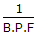# Mechanical Engineering - Heat Transfer, Refrigeration and Air Conditioning

### Exercise :: Heat Transfer, Refrigeration and Air Conditioning - Section 7

26.

In case of sensible heating of air, the coil efficiency is given by (where B.P.F. = By-pass factor)

 A. B.P.F. - 1 B. 1 - B.P.F. C.D. 1 + B.RF.

Explanation:

No answer description available for this question. Let us discuss.

27.

The coefficient of performance (C.O.P.) is the reciprocal of the efficiency of a heat engine.

 A. True B. False

Explanation:

No answer description available for this question. Let us discuss.

28.

The wet bulb temperature at 100% relative humidity is __________ dry bulb temperature.

 A. same as B. lower than C. higher than

Explanation:

No answer description available for this question. Let us discuss.

29.

The freezing point of sulphur dioxide is

 A. -56.6°C B. -75.2°C C. -77.7°C D. -135.8°C

Explanation:

No answer description available for this question. Let us discuss.

30.

The ratio of the energy absorbed by the body to the total energy falling on it, is called

 A. absorptive power B. emissive power C. emissivity D. none of these